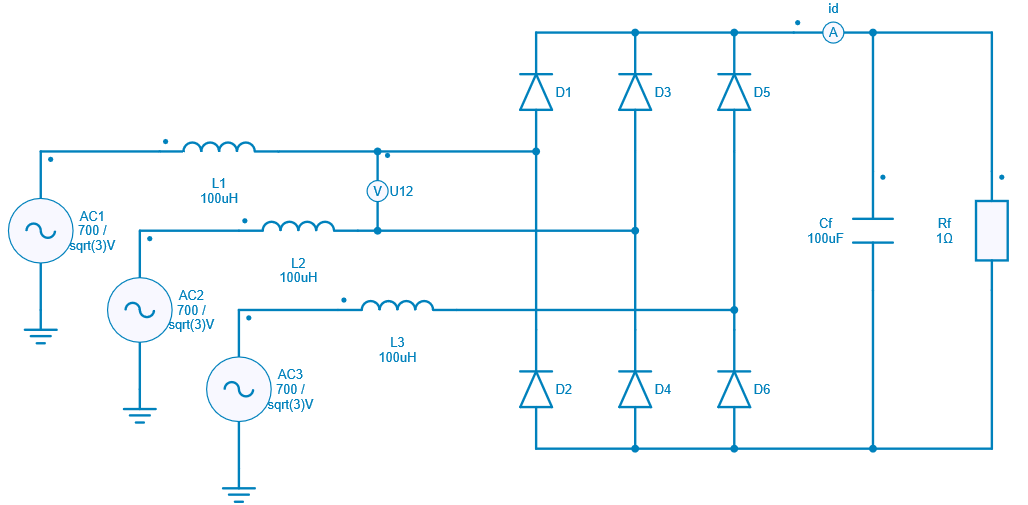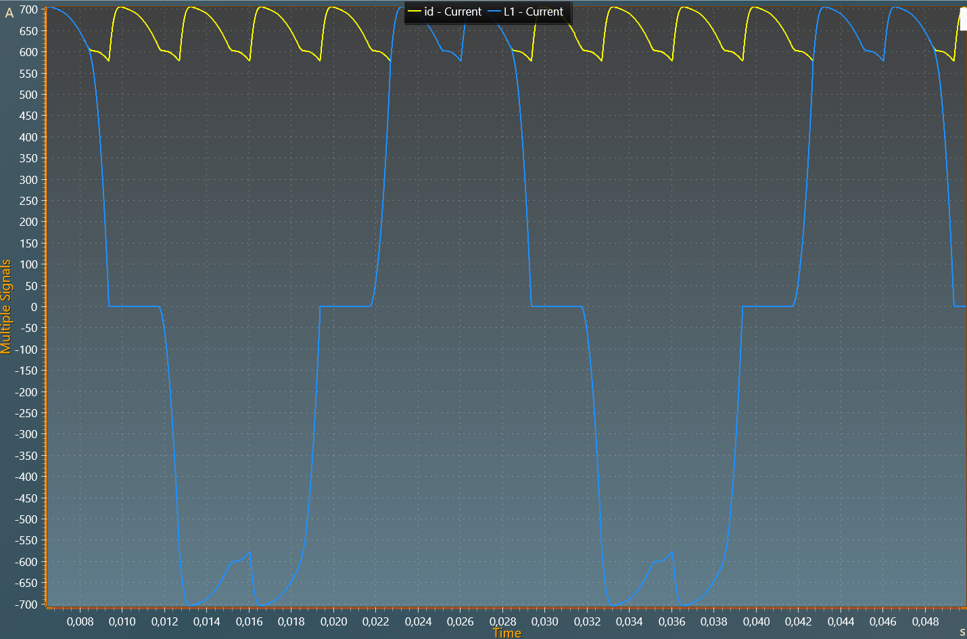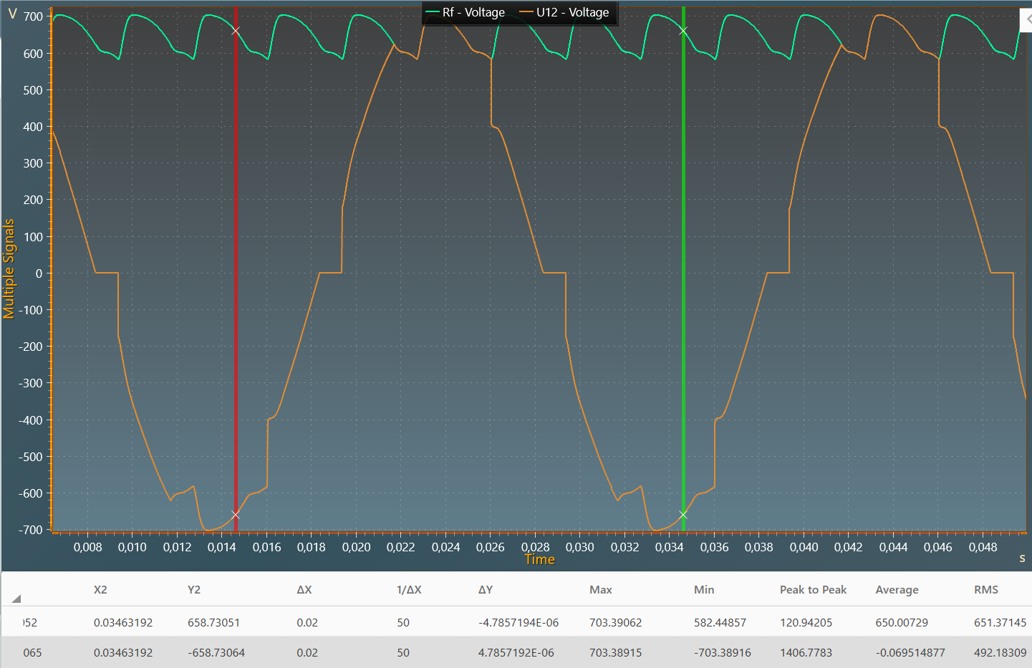Resources
• Tutorials >
• Application Examples
• Basic >
• PS and Drives >

# AC-DC 3-phase Diode Bridge with AC-side inductance

This example shows an AC-DC 3-phase full-wave diode bridge with:

• an inductive AC source with:
• a 3-phase voltage source of around 285 VRMS and a frequency of 50 Hz,
• a 3-phase inductor of 100 µH,
• a capacitive load with a DC capacitor of 100 µF,

With the simulated waveforms it underlines the behaviour of the diode bridge commutation with an inductive AC source.

## Electrical Model

The schematic below shows the electrical model considered. Diodes are ideal components.## Simulation

The figure below shows the AC input and DC output currents.The figure below shows the AC input and DC output voltages. A measurement of the DC voltage average value has been performed usinf scope cursors on a AC period (20 ms) and gives a value of around 650 V.